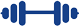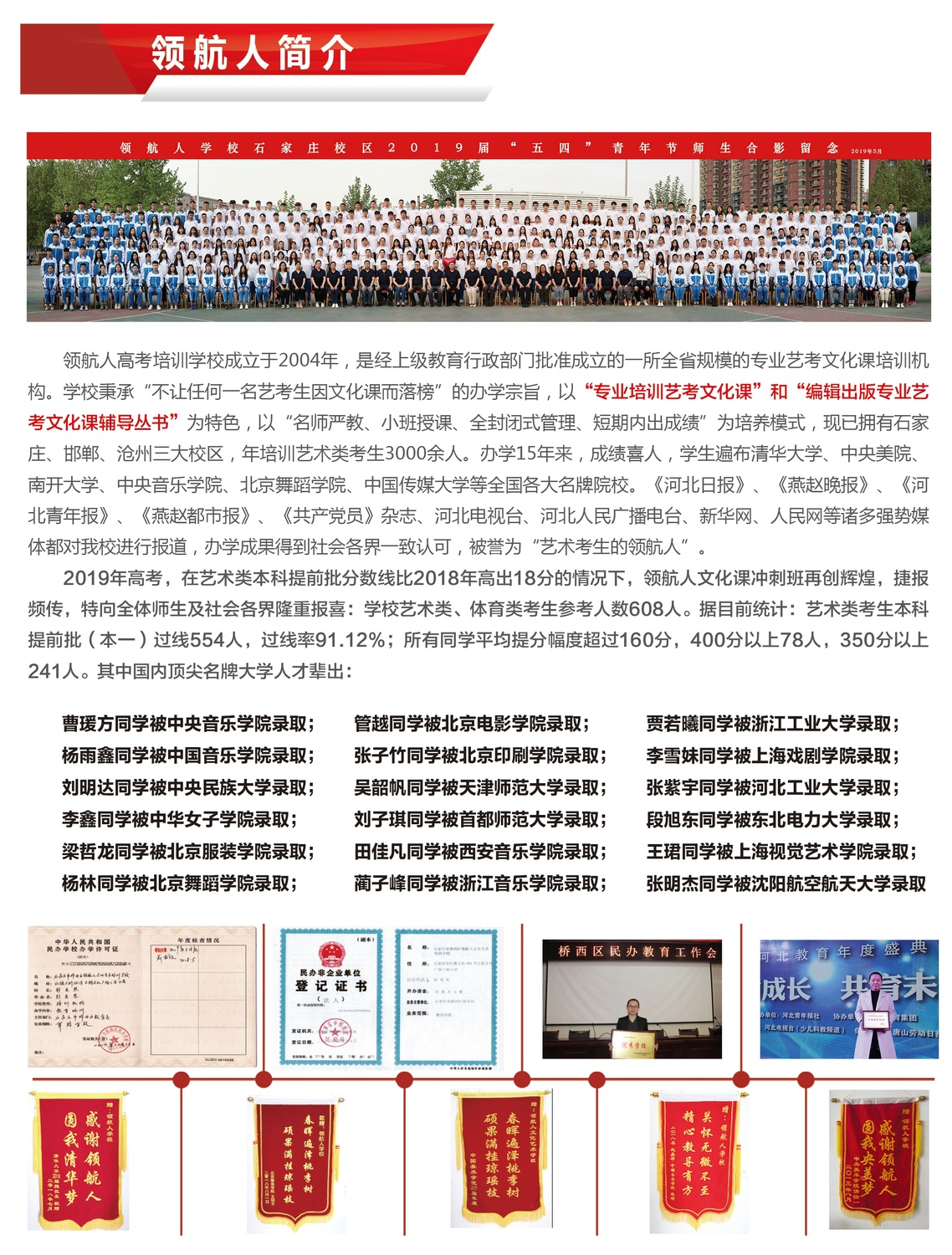# 数学技巧丨50个公式，50种快速做题方法！赶快来看！！

1 . 适用条件

[直线过焦点]，必有ecosA=(x-1)/(x+1)，其中A为直线与焦点所在轴夹角，是锐角。x为分离比，必须大于1。

2 . 函数的周期性问题(记忆三个)

(1)若f(x)=-f(x+k)，则T=2k;

(2)若f(x)=m/(x+k)(m不为0)，则T=2k;

(3)若f(x)=f(x+k)+f(x-k)，则T=6k。

3 . 关于对称问题(无数人搞不懂的问题)总结如下

(1)若在R上(下同)满足：f(a+x)=f(b-x)恒成立，对称轴为x=(a+b)/2

(2)函数y=f(a+x)与y=f(b-x)的图像关于x=(b-a)/2对称;

(3)若f(a+x)+f(a-x)=2b，则f(x)图像关于(a，b)中心对称

4 . 函数奇偶性

(1)对于属于R上的奇函数有f(0)=0;

(2)对于含参函数，奇函数没有偶次方项，偶函数没有奇次方项

(3)奇偶性作用不大，一般用于选择填空

5 . 数列爆强定律

(1)等差数列中：S奇=na中，例如S13=13a7(13和7为下角标);

(2)等差数列中：S(n)、S(2n)-S(n)、S(3n)-S(2n)成等差

(3)等比数列中，上述2中各项在公比不为负一时成等比，在q=-1时，未必成立

(4)等比数列爆强公式：S(n+m)=S(m)+q²mS(n)可以迅速求q

6 . 数列的终极利器，特征根方程

a1已知，那么特征根x=q/(1-p)，则数列通项公式为an=(a1-x)p²(n-1)+x，这是一阶特征根方程的运用。

7 . 函数详解补充

1、复合函数奇偶性：内偶则偶，内奇同外

2、复合函数单调性：同增异减

3、重点知识关于三次函数：恐怕没有多少人知道三次函数曲线其实是中心对称图形。

8 . 常用数列bn=n×(2²n)求和Sn=(n-1)×(2²(n+1))+2记忆方法

9 . 适用于标准方程(焦点在x轴)爆强公式

k椭=-{(b²)xo｝/{(a²)yo｝k双={(b²)xo｝/{(a²)yo｝k抛=p/yo

10 . 强烈推荐一个两直线垂直或平行的必杀技11 . 经典中的经典

12 . 爆强△面积公式

S=1/2∣mq-np∣其中向量AB=(m，n)，向量BC=(p，q)

13 .   你知道吗?空间立体几何中：以下命题均错

(1)空间中不同三点确定一个平面

(2)垂直同一直线的两直线平行

(3)两组对边分别相等的四边形是平行四边形

(4)如果一条直线与平面内无数条直线垂直，则直线垂直平面

(5)有两个面互相平行，其余各面都是平行四边形的几何体是棱柱

(6)有一个面是多边形，其余各面都是三角形的几何体都是棱锥

14 . 一个小知识点

15 . 求f(x)=∣x-1∣+∣x-2∣+∣x-3∣+…+∣x-n∣(n为正整数)的最小值

16 . √〔(a²+b²)〕/2≥(a+b)/2≥√ab≥2ab/(a+b)(a、b为正数，是统一定义域)

17 . 椭圆中焦点三角形面积公式

S=b²tan(A/2)在双曲线中：S=b²/tan(A/2)

18 . 爆强定理

(1)A为线线夹角

(2)A为线面夹角(但是公式中cos换成sin)

(3)A为面面夹角注：以上角范围均为[0，派/2]。

19 . 爆强公式

1²+2²+3²+…+n²=1/6(n)(n+1)(2n+1);1²3+2²3+3²3+…+n²3=1/4(n²)(n+1)²

20 . 爆强切线方程记忆方法21 .   爆强定理

(a+b+c)²n的展开式[合并之后]的项数为：Cn+22，n+2在下，2在上

22 .   转化思想

23 . 对于y²=2px

(题目的意思就是弦AB过焦点，CD过焦点，且AB垂直于CD)

24 . 关于一个重要绝对值不等式的介绍爆强

∣|a|-|b|∣≤∣a±b∣≤∣a∣+∣b∣

25 . 关于解决证明含ln的不等式的一种思路

an=1×1/n=矩形面积>曲线下面积=bn。当然前面要证明1>ln2。

26 . 爆强简洁公式

27 .   说明一个易错点

28 . 离心率爆强公式

e=sinA/(sinM+sinN)

29 . 椭圆的参数方程也是一个很好的东西，它可以解决一些最值问题。

30 . 仅供有能力的童鞋参考的爆强公式

sinθ+sinφ=2sin[(θ+φ)/2]cos[(θ-φ)/2]sinθ-sinφ=2cos[(θ+φ)/2]sin[(θ-φ)/2]cosθ+cosφ=2cos[(θ+φ)/2]cos[(θ-φ)/2]cosθ-cosφ=-2sin[(θ+φ)/2]sin[(θ-φ)/2]

sinαsinβ=[cos(α-β)-cos(α+β)]/2cosαcosβ=[cos(α+β)+cos(α-β)]/2sinαcosβ=[sin(α+β)+sin(α-β)]/2cosαsinβ=[sin(α+β)-sin(α-β)]/231   . 爆强定理

32 . 三角形垂心爆强定理

(1)向量OH=向量OA+向量OB+向量OC(O为三角形外心，H为垂心)

(2)若三角形的三个顶点都在函数y=1/x的图象上，则它的垂心也在这个函数图象上。

33 . 维维安尼定理(不是很重要(仅供娱乐))

34 . 爆强思路

35 . 常用结论

O为原点，连接AO.BO。必有角AOB=90度

36 . 爆强公式

ln(x+1)≤x(x>-1)该式能有效解决不等式的证明问题。

37 . 函数y=(sinx)/x是偶函数

38 . 函数

y=(lnx)/x在(0，e)上单调递增，在(e，+无穷)上单调递减。

39 . 几个数学易错点

(1)f`(x)<0是函数在定义域内单调递减的充分不必要条件

(2)研究函数奇偶性时，忽略最开始的也是最重要的一步：考虑定义域是否关于原点对称

(3)不等式的运用过程中，千万要考虑"="号是否取到

(4)研究数列问题不考虑分项，就是说有时第一项并不符合通项公式，所以应当极度注意：数列问题一定要考虑是否需要分项!

40 . 提高计算能力五步曲

(1)扔掉计算器

(2)仔细审题(提倡看题慢，解题快)，要知道没有看清楚题目，你算多少都没用

(3)熟记常用数据，掌握一些速算技

(4)加强心算、估算能力

(5)检验41 . 一个美妙的公式

42 . 函数

①函数单调性的含义：大多数同学都知道若函数在区间D上单调，则函数值随着自变量的增大(减小)而增大(减小)，但有些意思可能有些人还不是很清楚，若函数在D上单调，则函数必连续(分段函数另当别论)这也说明了为什么不能说y=tanx在定义域内单调递增，因为它的图像被无穷多条渐近线挡住，换而言之，不连续.还有，如果函数在D上单调，则函数在D上y与x一一对应.这个可以用来解一些方程.至于例子不举了

②函数周期性：这里主要总结一些函数方程式所要表达的周期设f(x)为R上的函数，对任意x∈R

(1)f(a±x)=f(b±x)T=(b-a)(加绝对值，下同)

(2)f(a±x)=-f(b±x)T=2(b-a)

(3)f(x-a)+f(x+a)=f(x)T=6a

(4)设T≠0，有f(x+T)=M[f(x)]其中M(x)满足M[M(x)]=x,且M(x)≠x则函数的周期为2

43 . 奇偶函数概念的推广

(1)对于函数f(x)，若存在常数a，使得f(a-x)=f(a+x)，则称f(x)为广义(Ⅰ)型偶函数，且当有两个相异实数a，b满足时，f(x)为周期函数T=2(b-a)

(2)若f(a-x)=-f(a+x)，则f(x)是广义(Ⅰ)型奇函数，当有两个相异实数a，b满足时，f(x)为周期函数T=2(b-a)

(3)有两个实数a，b满足广义奇偶函数的方程式时，就称f(x)是广义(Ⅱ)型的奇，偶函数.且若f(x)是广义(Ⅱ)型偶函数，那么当f在[a+b/2，∞)上为增函数时，有f(x1)<f(x2)等价于绝对值x1-(a+b p="" <="" 2)<绝对值x2-(a+b)="">

44 . 函数对称性

(1)若f(x)满足f(a+x)+f(b-x)=c则函数关于(a+b/2，c/2)成中心对称

(2)若f(x)满足f(a+x)=f(b-x)则函数关于直线x=a+b/2成轴对称

(1)若f(xy)=f(x)+f(y)(x>0,y>0),则f(x)=㏒ax

(2)若f(xy)=f(x)f(y)(x>0,y>0),则f(x)=x²u(u由初值给出)

(3)f(x+y)=f(x)f(y)则f(x)=a²x

(4)若f(x+y)=f(x)+f(y)+kxy,则f(x)=ax2+bx(5)若f(x+y)+f(x-y)=2f(x),则f(x)=ax+b特别的若f(x)+f(y)=f(x+y)，则f(x)=kx

45 . 与三角形有关的定理或结论中学数学平面几何最基本的图形就是三角形

①正切定理(我自己取的，因为不知道名字)：在非Rt△中，有tanA+tanB+tanC=tanAtanBtanC

②任意三角形射影定理(又称第一余弦定理)：

a=bcosC+ccosB;b=ccosA+acosC;c=acosB+bcosA

③任意三角形内切圆半径r=2S/a+b+c(S为面积)，外接圆半径应该都知道了吧

④梅涅劳斯定理：设A1,B1，C1分别是△ABC三边BC，CA，AB所在直线的上的点，则A1，B1，C1共线的充要条件是CB1/B1A·BA1/A1C·AC1/C1B=1

44 . 易错点

(1)函数的各类性质综合运用不灵活，比如奇偶性与单调性常用来配合解决抽象函数不等式问题;

(2)三角函数恒等变换不清楚，诱导公式不迅捷。

45 . 易错点

(3)忽略三角函数中的有界性，三角形中角度的限定，比如一个三角形中，不可能同时出现两个角的正切值为负

(4)三角的平移变换不清晰，说明：由y=sinx变成y=sinwx的步骤是将横坐标变成原来的1/∣w∣倍

46 . 易错点

(5)数列求和中，常常使用的错位相减总是粗心算错

(6)数列中常用变形公式不清楚，如：an=1/[n(n+2)]的求和保留四项

47 . 易错点

(7)数列未考虑a1是否符合根据sn-sn-1求得的通项公式;

(8)数列并不是简单的全体实数函数，即注意求导研究数列的最值问题过程中是否取到问题

48 . 易错点

(9)向量的运算不完全等价于代数运算;

(10)在求向量的模运算过程中平方之后，忘记开方。

(11)复数的几何意义不清晰

49 . 关于辅助角公式

asint+bcost=[√(a²+b²)]sin(t+m)其中tanm=b/a[条件：a>0]

50 . A、B为椭圆x²/a²+y²/b²=1上任意两点。若OA垂直OB，则有1/∣OA∣²+1/∣OB∣²=1/a²+1/b²